Chapter 3, Problem 3.90EP### Organic And Biological Chemistry

7th Edition
STOKER + 1 other
ISBN: 9781305081079

#### Solutions

Chapter
Section### Organic And Biological Chemistry

7th Edition
STOKER + 1 other
ISBN: 9781305081079
Textbook Problem

# What is the difference in meaning associated with each of the following pairs of notations? a. R—O—R and R—O—H b. Ar—O—R and Ar—O—Ar c. Ar—O—H and Ar—O—R d. R—O—R and Ar—O—Ar

(a)

Interpretation Introduction

Interpretation:

The difference in meaning that is associated with the given pair of notations has to be given.

Concept Introduction:

Ether is an organic compound.  In ether, an oxygen atom is bonded to two carbon atoms by a single bond.  The groups that are attached to the carbon atom by a single bond may be alkyl, aryl, or cycloalkyl groups.  The functional group of ether is COC.  The generalized formula of ether can be given as,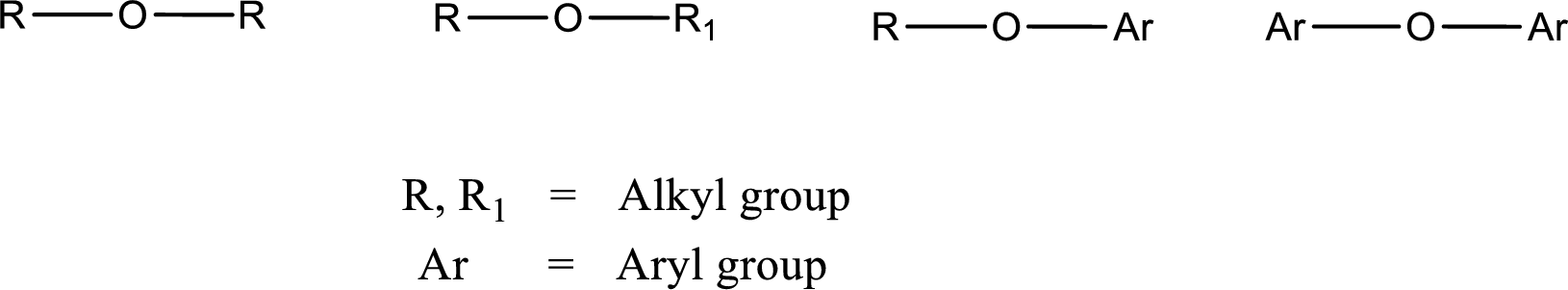Alcohol is a compound in which a hydrocarbon group is bonded to a hydroxyl group.  The generalized formula for alcohol can be given as,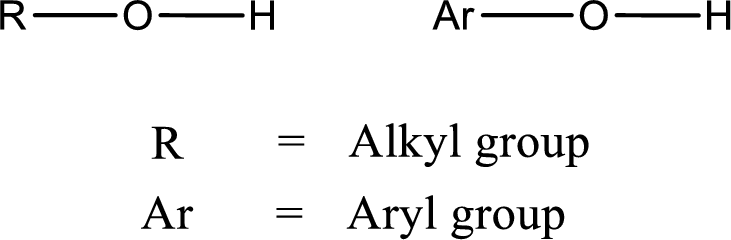Explanation

Ethers are compound that have an oxygen atom that is bonded to two carbon atoms through a single bonds.  The carbon atoms may be from alkyl group, aryl group, or cycloalkyl group.  In simple words, the oxygen atom that is bonded to two hydrocarbon groups through a single bond is known as ether.

Alcohols are compounds that contain hydroxyl group bonded to a hydrocarbon.  In simple words, if a hydrocarbon group and hydrogen atom is attached to an oxygen atom through single bonds is known as alcohol.

Given structural notations are ROR and ROH

(b)

Interpretation Introduction

Interpretation:

The difference in meaning that is associated with the given pair of notations has to be given.

Concept Introduction:

Ether is an organic compound.  In ether, an oxygen atom is bonded to two carbon atoms by a single bond.  The groups that are attached to the carbon atom by a single bond may be alkyl, aryl, or cycloalkyl groups.  The functional group of ether is COC.  The generalized formula of ether can be given as,Alcohol is a compound in which a hydrocarbon group is bonded to a hydroxyl group.  The generalized formula for alcohol can be given as,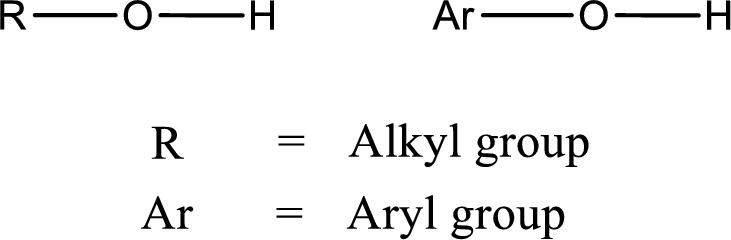(c)

Interpretation Introduction

Interpretation:

The difference in meaning that is associated with the given pair of notations has to be given.

Concept Introduction:

Ether is an organic compound.  In ether, an oxygen atom is bonded to two carbon atoms by a single bond.  The groups that are attached to the carbon atom by a single bond may be alkyl, aryl, or cycloalkyl groups.  The functional group of ether is COC.  The generalized formula of ether can be given as,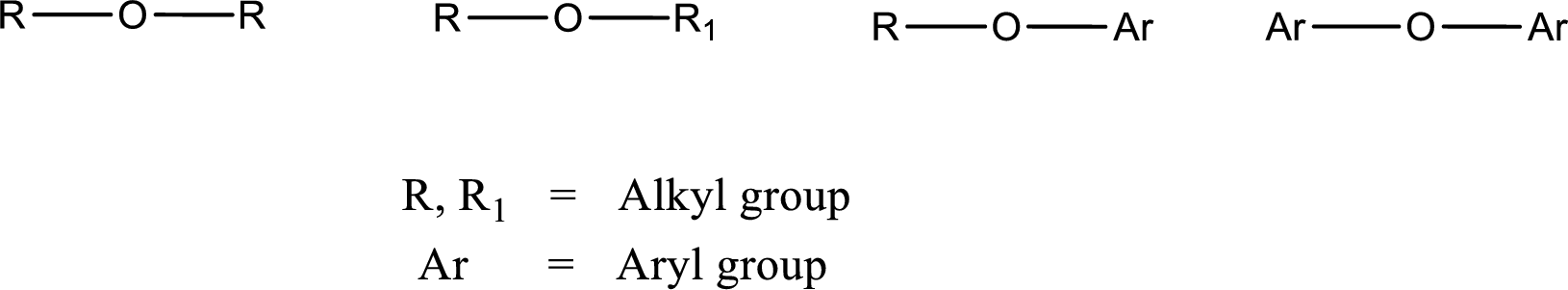Alcohol is a compound in which a hydrocarbon group is bonded to a hydroxyl group.  The generalized formula for alcohol can be given as,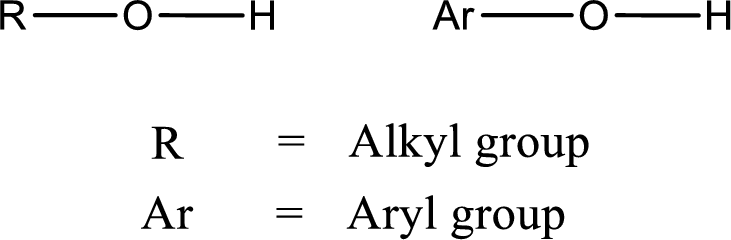(d)

Interpretation Introduction

Interpretation:

The difference in meaning that is associated with the given pair of notations has to be given.

Concept Introduction:

Ether is an organic compound.  In ether, an oxygen atom is bonded to two carbon atoms by a single bond.  The groups that are attached to the carbon atom by a single bond may be alkyl, aryl, or cycloalkyl groups.  The functional group of ether is COC.  The generalized formula of ether can be given as,Alcohol is a compound in which a hydrocarbon group is bonded to a hydroxyl group.  The generalized formula for alcohol can be given as,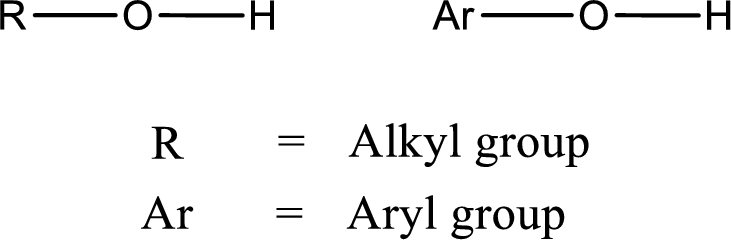### Still sussing out bartleby?

Check out a sample textbook solution.

See a sample solution

#### The Solution to Your Study Problems

Bartleby provides explanations to thousands of textbook problems written by our experts, many with advanced degrees!

Get Started